# ELEMENTARY GAS LAWS GAS LAWS INTRODUCTION Gas laws

• Slides: 20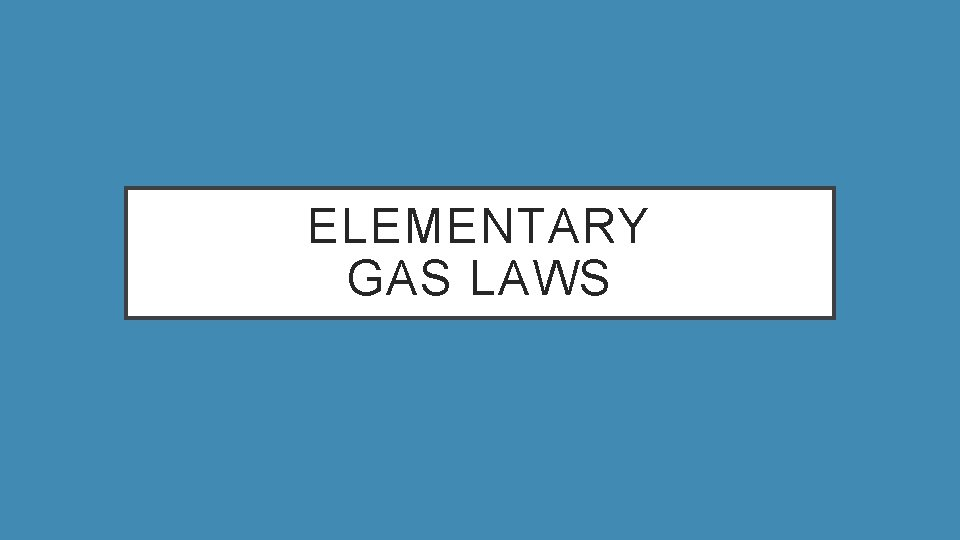ELEMENTARY GAS LAWS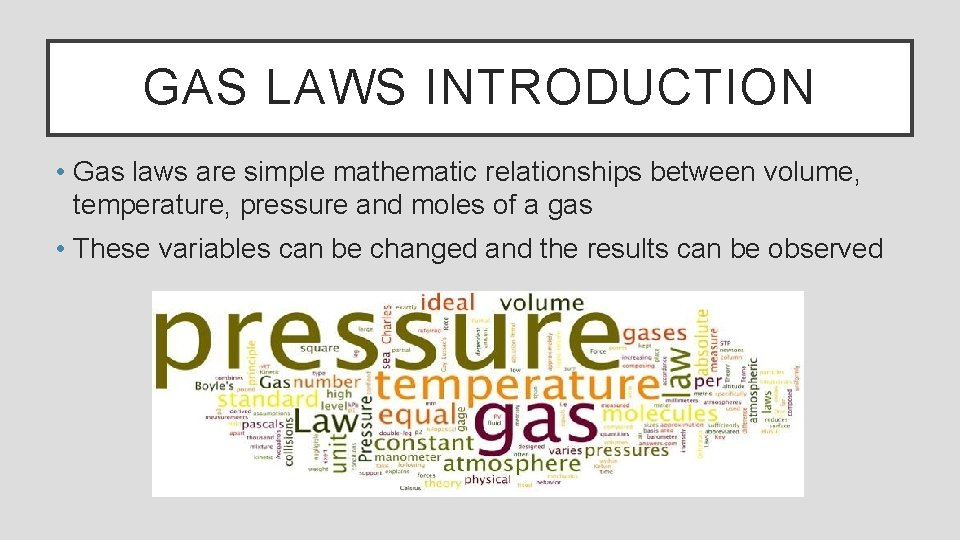GAS LAWS INTRODUCTION • Gas laws are simple mathematic relationships between volume, temperature, pressure and moles of a gas • These variables can be changed and the results can be observed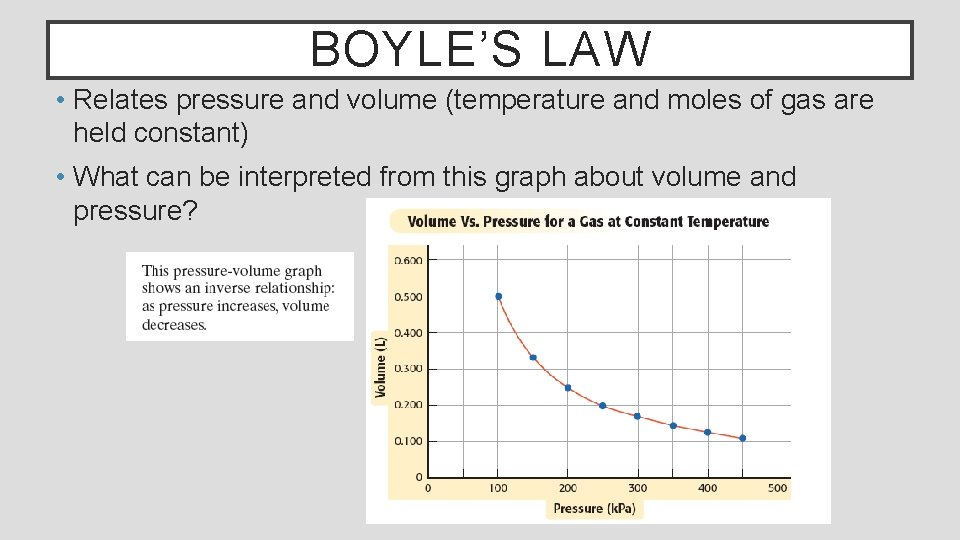BOYLE’S LAW • Relates pressure and volume (temperature and moles of gas are held constant) • What can be interpreted from this graph about volume and pressure?BOYLE’S LAW Boyle’s law states that the volume of a fixed amount of gas (moles) varies inversely with the pressure at a constant temperature Mathematically, his law is expressed as Where V is volume, P is pressure and k is a constant Boyle’s law can be used to compare changing pressure/volume conditions for a gas Where P 1 and V 1 are initial conditions and P 2 and V 2 are new conditionsEXAMPLE A sample of oxygen gas has a volume of 150. m. L when its pressure is 0. 947 atm. What will the volume of the gas be at a pressure of 0. 987 atm if the temperature remains constant? The final volume would be 144 m. L of O 2LET’S PRACTICE USING BOYLE’S LAW Page 559 #1, 2CHARLES’ LAW • Relates volume and temperature (pressure and moles of gas are held constant) • What can be interpreted from this graph about volume and temperature?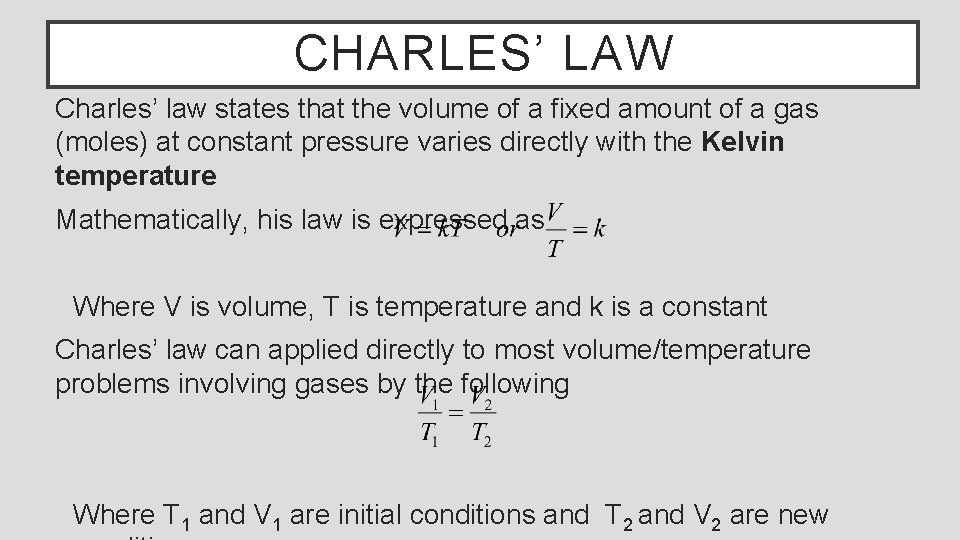CHARLES’ LAW Charles’ law states that the volume of a fixed amount of a gas (moles) at constant pressure varies directly with the Kelvin temperature Mathematically, his law is expressed as Where V is volume, T is temperature and k is a constant Charles’ law can applied directly to most volume/temperature problems involving gases by the following Where T 1 and V 1 are initial conditions and T 2 and V 2 are new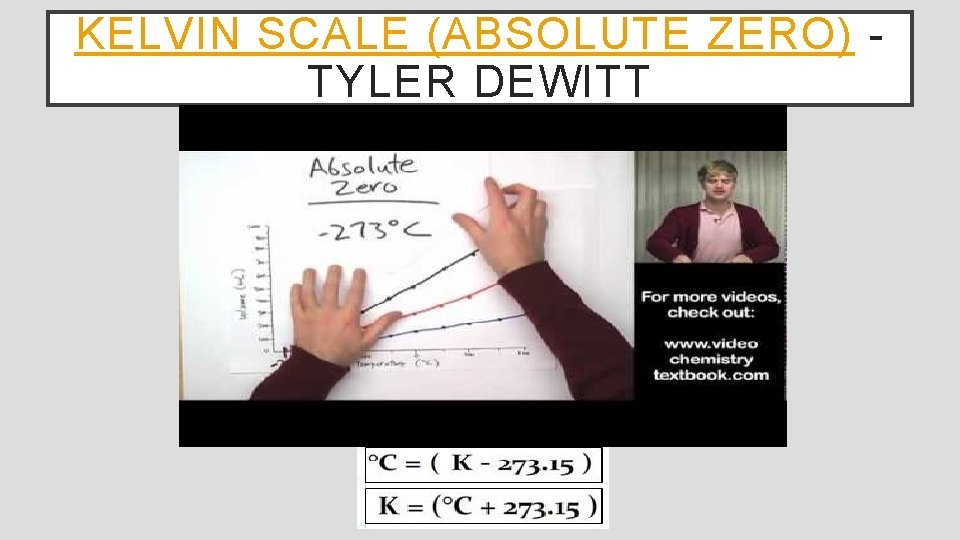KELVIN SCALE (ABSOLUTE ZERO) TYLER DEWITT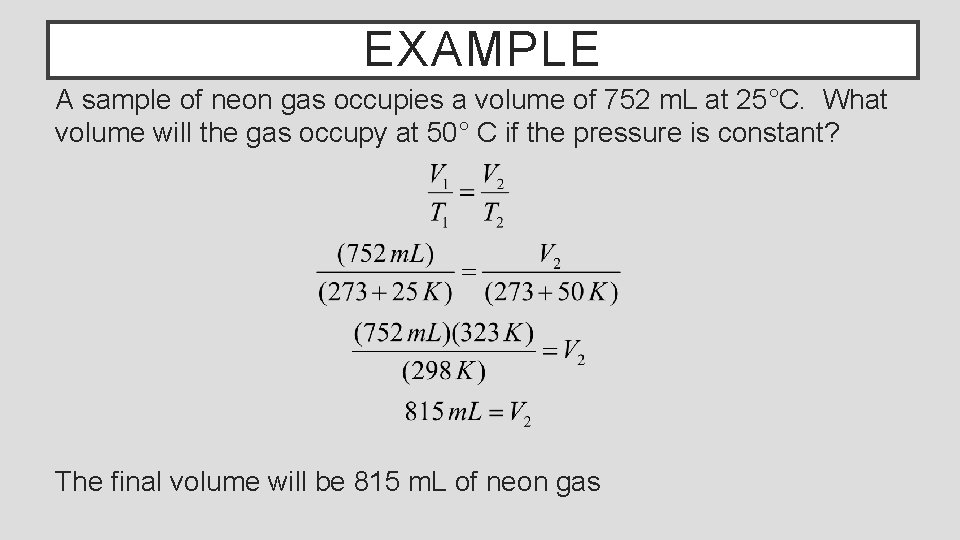EXAMPLE A sample of neon gas occupies a volume of 752 m. L at 25°C. What volume will the gas occupy at 50° C if the pressure is constant? The final volume will be 815 m. L of neon gasLET’S PRACTICE USING CHARLES’ LAW Page 552 #1, 2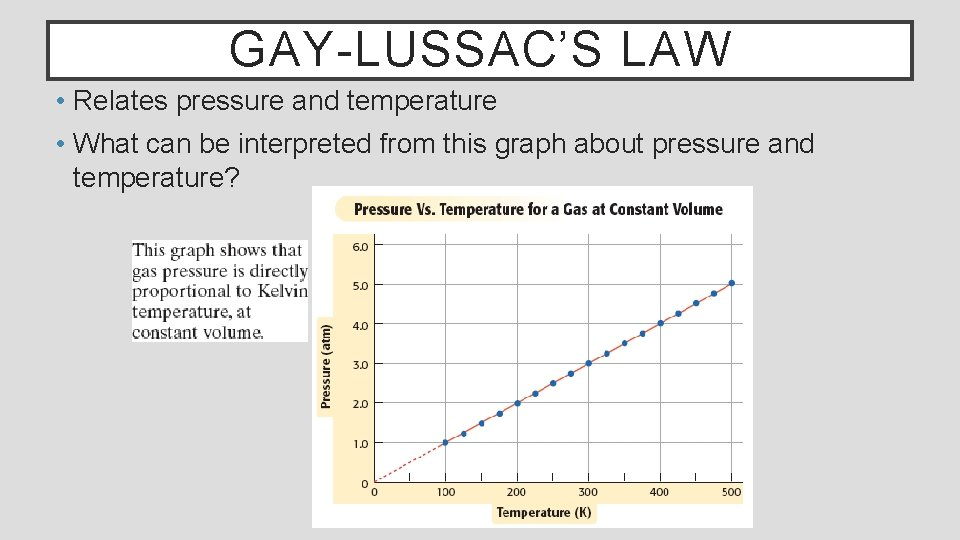GAY-LUSSAC’S LAW • Relates pressure and temperature • What can be interpreted from this graph about pressure and temperature?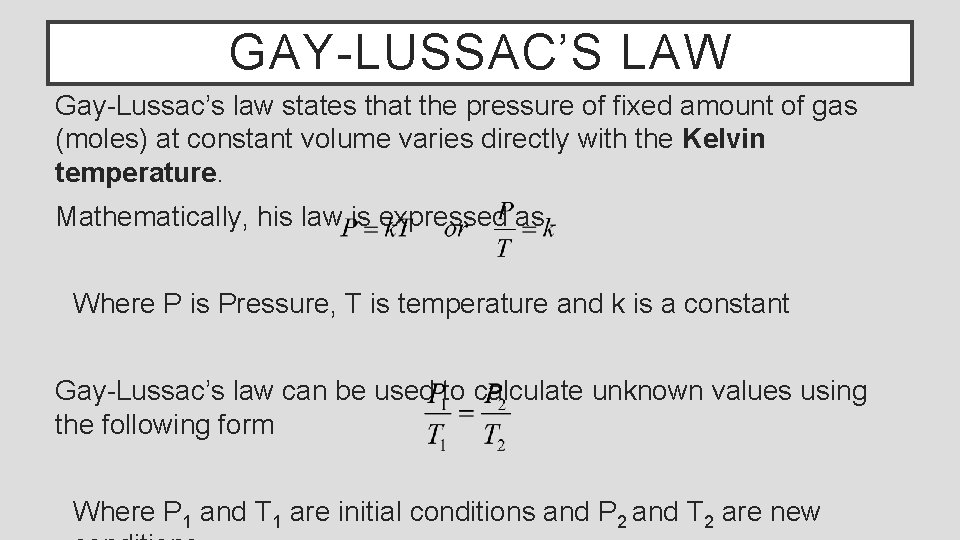GAY-LUSSAC’S LAW Gay-Lussac’s law states that the pressure of fixed amount of gas (moles) at constant volume varies directly with the Kelvin temperature. Mathematically, his law is expressed as Where P is Pressure, T is temperature and k is a constant Gay-Lussac’s law can be used to calculate unknown values using the following form Where P 1 and T 1 are initial conditions and P 2 and T 2 are newEXAMPLE The gas in an aerosol can is at a pressure of 3. 00 atm at 25°C. Directions on the can warn the user not to keep the can in a place where the temperature exceeds 52°C. What would the gas pressure be in the can at 52° C? The final pressure would be 3. 27 atm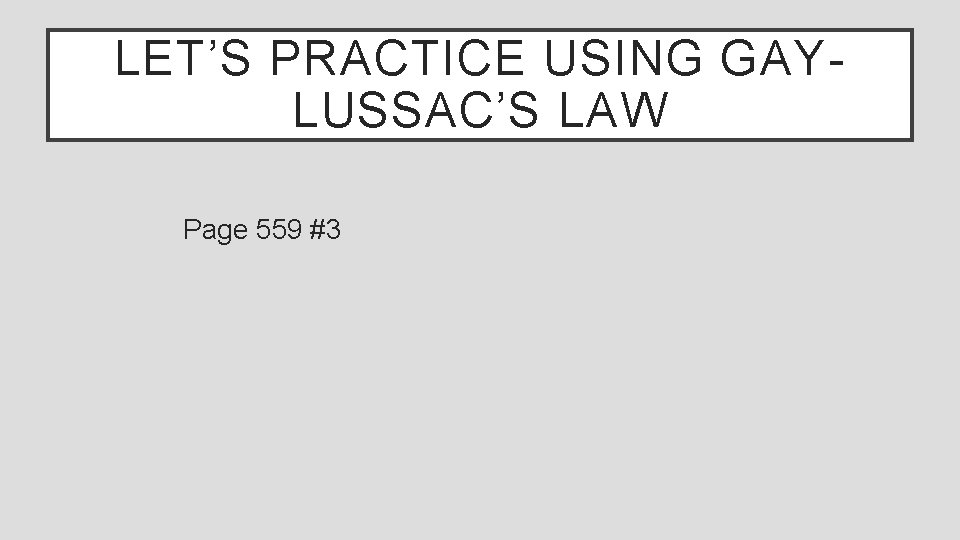LET’S PRACTICE USING GAYLUSSAC’S LAW Page 559 #3COMBINED GAS LAW A gas sample often undergoes changes in temperature, pressure and volume at the same time. When this happens, Boyle’s, Charles’s and Gay-Lussac laws must be combined The combined gas law expresses the relationship between pressure, volume and temperature of a fixed amount of gas (moles) Mathematically this is expressed as Where P is pressure, V is volume, T is temperature and k is a constant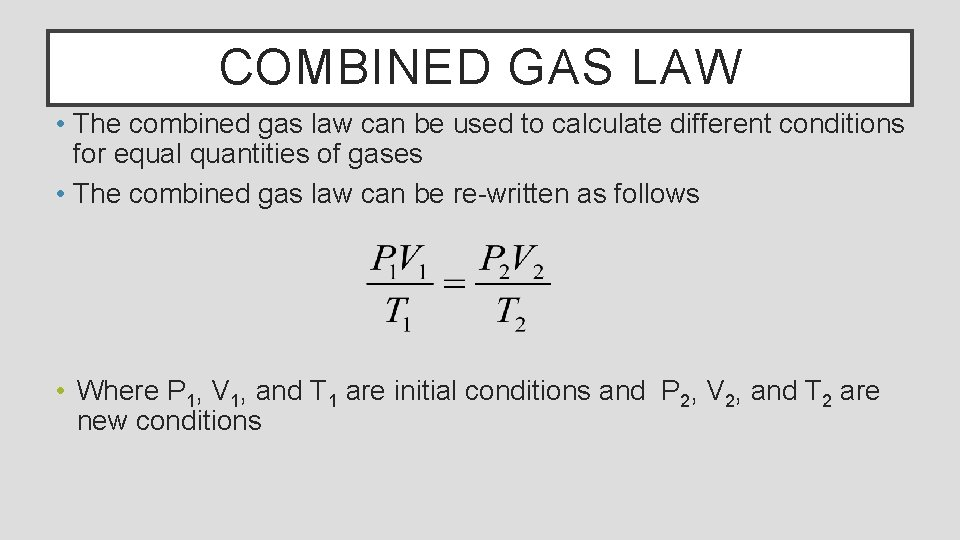COMBINED GAS LAW • The combined gas law can be used to calculate different conditions for equal quantities of gases • The combined gas law can be re-written as follows • Where P 1, V 1, and T 1 are initial conditions and P 2, V 2, and T 2 are new conditionsEXAMPLE A helium filled balloon has a volume of 50. 0 L at 25°C and 1. 08 atm. What volume will it have at 0. 855 atm and 10. °C? The final volume will be 60. 0 L of Helium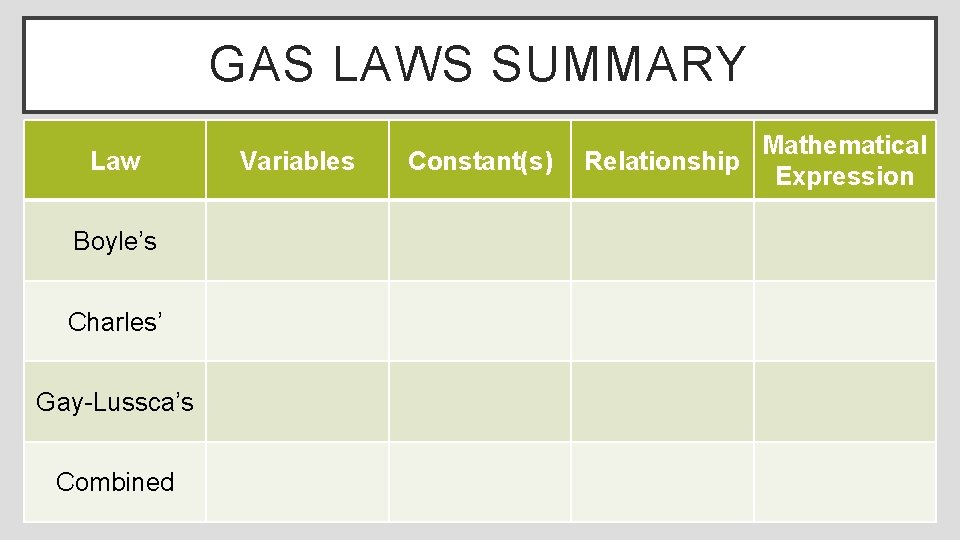GAS LAWS SUMMARY Law Boyle’s Charles’ Gay-Lussca’s Combined Variables Constant(s) Mathematical Relationship Expression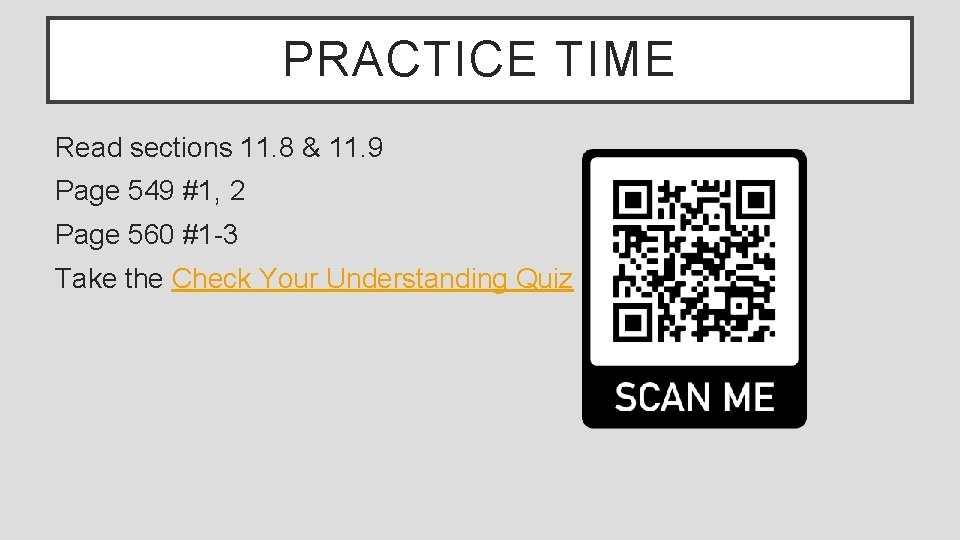PRACTICE TIME Read sections 11. 8 & 11. 9 Page 549 #1, 2 Page 560 #1 -3 Take the Check Your Understanding Quiz